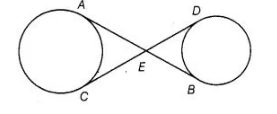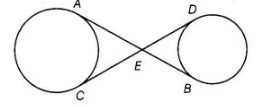# In figure, common tangents AB and CD

Question:

In figure, common tangents AB and CD to two circles intersect at E. Prove that AB = CD.Solution:

Given Common tangents AB and CD to two circles intersecting at E.

To prove                                           AB = CDProof $E A=E C$ $\ldots(1)$

[the lengths of tanoents drawn from an internal point to a circle are equal]

$E B=E D$ ....(ii)

On adding Eqs. (i) and (ii), we get

$E A+E B=E C+E D$

$\Rightarrow$ $A B=C D$  Hence proved.